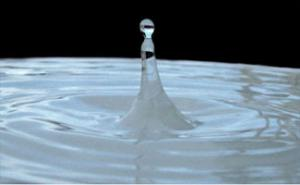# What is Scientific Notation?Source: Water droplet, Saperaud, Wikimedia Commons

Science often uses very small and very large numbers, which can lead to confusion.

Consider a water molecule. The length of one single water molecule is about 0.000000000278 meters. That's a very small number.

In 1 mL of water there are about 33400000000000000000000 molecules. That's a very large number.

Those very small and very large numbers can be difficult to work with as well as inconvenient to write. Scientific notation is a way to make calculations using these numbers easier.

In scientific notation, a number is written as the product of two numbers:

$\mathbf{a}\mathbf{§#xA0;}{\mathbf{coefficient}}\mathbf{§#xA0;}\mathbf{and}\mathbf{§#xA0;}{{\mathbf{10}}}^{\mathbf{raised}\mathbf{§#xA0;}\mathbf{to}\mathbf{§#xA0;}\mathbf{a}\mathbf{§#xA0;}\mathbf{power}}$

For example, in scientific notation

$\mathbf{2555}\mathbf{§#xA0;}\mathbf{=}{\mathbf{§#xA0;}}{\mathbf{2}}{\mathbf{.}}{\mathbf{555}}§#xA0;\mathbf{§#xD7;}§#xA0;{{\mathbf{10}}}^{\mathbf{3}}$

The coefficient is always a number greater than or equal to one and less than or equal to 10.

$\mathbf{1}\mathbf{§#xA0;}\mathbf{§#x2264;}\mathbf{§#xA0;}{\mathbf{coefficient}}\mathbf{§#xA0;}\mathbf{§#x2265;}\mathbf{§#xA0;}\mathbf{10}$

In this case the coefficient is 2.555.

The power of 10 corresponds to the number of places the decimal moved. A positive power of 10 would indicate the standard form is greater than zero (decimal was moved to the left.) A negative power of 10 would indicate a number less than zero (decimal was moved to the right.)

In the example above, the number in standard form was greater than zero so the decimal moved three places to the left; therefore, the power of 10 or exponent is three.

# Writing Numbers in Scientific Notation

Let’s look at some examples of writing numbers into scientific notation. Watch this video to learn more about writing numbers in scientific notation.

Source: Scientific Notation, mahalodotcom, You Tube

Now let’s practice writing numbers in scientific notation.

# Converting Numbers from Scientific Notation

When you convert numbers from scientific notation, the steps are the opposite of writing numbers in scientific notation. Watch this video to learn how to convert numbers from scientific notation.

Source: How to go from scientific notation to standard form, YourTutorOnline, YouTube

# Adding and Subtracting Numbers in Scientific Notation

Sometimes you may need to add or subtract numbers that are expressed in scientific notation. Watch this video to learn how!